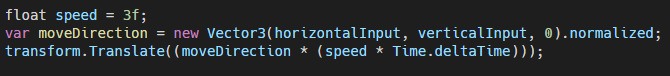# Simple Player Movement in Unity

Unity3D 2 min read , May 3, 2021

Some of the most basic player movement is moving off WASD, Arrow Keys, or with a game controller’s Left stick. In Unity3d this is fairly easy but still trips a few people up.

## How Unity’s Input System Works

Unity does a lot of the hard work for us, we don’t need to “know” how windows or mac or our phone handles input as Unity has a built-in InputManager. They even give us default input controls to get started.

Simply open “Project Settings > Input Manager” and you can see it has several events already pre-defined including Horizontal and Vertical.

For basic moving input, we want Horizontal and Vertical.

## The Code

We simply have to apply a custom class such as Player.cs and ask the InputManager to get the current values we do that with the below code within our Update() function.

Now the way the values are stored are -1.0 float to 1.0 float and they’ll scale up/down from 0.

Now a common mistake many developers make is typing “horizontal” with a lower-case h or “vertical”. This causes no errors to appear, but always return zero for a value as to the InputManager it’s “a different setting”. You need to exactly match what’s in the Project settings > input manager.

## Using the Input to Move

From here we need to do some Vector math and code.
So we want to:

1. Translate the two float inputs into a Vector3 so we can work with it.
2. We will Normalize this Vector as we just want Direction, not the magnitude. Not doing this can make players move faster on diagonals.
3. Multiply it with a playerSpeed as the raw input is always between -1 and 1.
4. Then because we’re in Update() multiply by time.deltatime to modify for FrameRate
5. Then finally apply the position change to our object’s transform position

This looks like this in codeYou can see we “squished” 1 and 2 into one line, and 3, 4, 5 into another

## Why Normalize Vectors

Normalizing is done as we just want the “Direction” of the vector, and in Vector math we do this by ensuring all points in the vector adds up to 1.

So with us holding right and up (vertical=1, horzontal=1) Normalizing will give us (0.5, 0.5, 0) which 0.5+0.5+0 = 1 so when we multiply by our speed (speed=3) this would give us(1.5, 1.5, 0) for a Vector3 which also adds up to our speed 1.5+1.5+0=3.

While not doing this just gives us the magnitude of the vector which is (1.0, 1.0, 0) so if we multiply this vector by our speed of 3, we’d get (3, 3, 0) which is a total of 6 positional changes instead of 3 or “double” our speed!

## Player.cs

Fullcode of Player.cs

`using UnityEngine;public class Player : MonoBehaviour{    [Tooltip("Players movement speed")]    [SerializeField] float speed = 3f;    private void Update()    {       float horizontalInput = Input.GetAxis("Horizontal");       float verticalInput  = Input.GetAxis("Vertical");       var moveDirection = new Vector3(horizontalInput, verticalInput, 0).normalized;       transform.Translate((moveDirection * (speed * Time.deltaTime)));    }}`
Related stories